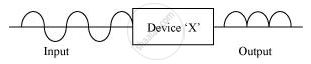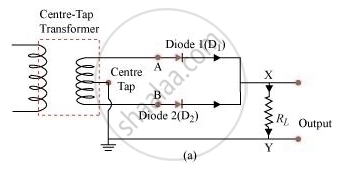# In the Figure Given Below the Input Waveform is Converted into the Output Waveform by a Device ‘X’. Name the Device and Draw Its Circuit Diagram. - Physics

#### Question

In the figure given below the input waveform is converted into the output waveform by a device ‘X’. Name the device and draw its circuit diagram.#### Solution

The device is a full-wave rectifier.

The circuit diagram of a full-wave rectifier is represented asIs there an error in this question or solution?